Documentation

### This is machine translation

Mouseover text to see original. Click the button below to return to the English version of the page.

Note: This page has been translated by MathWorks. Click here to see
To view all translated materials including this page, select Country from the country navigator on the bottom of this page.

# polarplot

Plot line in polar coordinates

## Syntax

``polarplot(theta,rho)``
``polarplot(theta,rho,LineSpec)``
``polarplot(theta1,rho1,...,thetaN,rhoN)``
``polarplot(theta1,rho1,LineSpec1,...,thetaN,rhoN,LineSpecN)``
``polarplot(rho)``
``polarplot(rho,LineSpec)``
``polarplot(Z)``
``polarplot(Z,LineSpec)``
``polarplot(___,Name,Value)``
``polarplot(pax,___)``
``p = polarplot(___)``

## Description

example

````polarplot(theta,rho)` plots a line in polar coordinates, with `theta` indicating the angle in radians and `rho` indicating the radius value for each point. The inputs must be vectors with equal length or matrices with equal size. If the inputs are matrices, then `polarplot` plots columns of `rho` versus columns of `theta`. Alternatively, one of the inputs can be a vector and the other a matrix as long as the vector is the same length as one dimension of the matrix.```

example

````polarplot(theta,rho,LineSpec)` sets the line style, marker symbol, and color for the line.```
````polarplot(theta1,rho1,...,thetaN,rhoN)` plots multiple `rho,theta` pairs.```
````polarplot(theta1,rho1,LineSpec1,...,thetaN,rhoN,LineSpecN)` specifies the line style, marker symbol, and color for each line.```

example

````polarplot(rho)` plots the radius values in `rho` at evenly spaced angles between 0 and 2π.```
````polarplot(rho,LineSpec)` sets the line style, marker symbol, and color for the line.```

example

````polarplot(Z)` plots the complex values in `Z`.```
````polarplot(Z,LineSpec)` sets the line style, marker symbol, and color for the line.```
````polarplot(___,Name,Value)` specifies properties of the chart line using one or more `Name,Value` pair arguments. The property settings apply to all the lines. You cannot specify different property values for different lines using `Name,Value` pairs.```
````polarplot(pax,___)` uses the `PolarAxes` object specified by `pax`, instead of the current axes.```

example

````p = polarplot(___)` returns one or more chart line objects. Use `p` to set properties of a specific chart line object after it is created. For a list of properties, see Line Properties.```

## Examples

collapse all

Plot a line in polar coordinates.

```theta = 0:0.01:2*pi; rho = sin(2*theta).*cos(2*theta); polarplot(theta,rho)```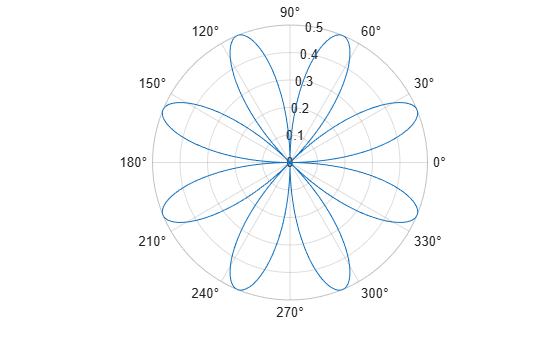Create the data to plot.

```theta = linspace(0,360,50); rho = 0.005*theta/10;```

Convert the values in `theta` from degrees to radians. Then, plot the data in polar coordinates.

```theta_radians = deg2rad(theta); polarplot(theta_radians,rho)```Plot two lines in polar coordinates. Use a dashed line for the second line.

```theta = linspace(0,6*pi); rho1 = theta/10; polarplot(theta,rho1) rho2 = theta/12; hold on polarplot(theta,rho2,'--') hold off```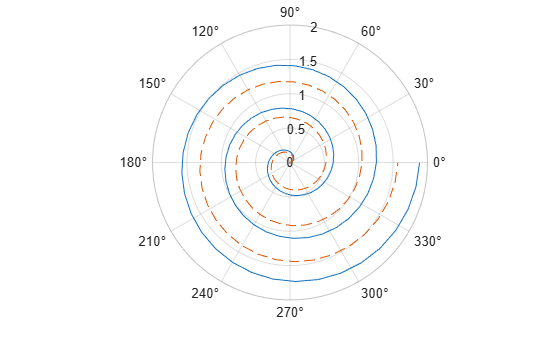Specify only the radius values, without specifying the angle values. `polarplot` plots the radius values at equally spaced angles that span from 0 to $2\pi$. Display a circle marker at each data point.

```rho = 10:5:70; polarplot(rho,'-o')```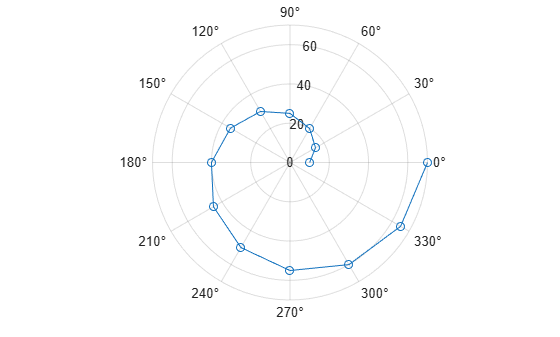Create a polar plot using negative radius values. By default, `polarplot` reflects negative values through the origin.

```theta = linspace(0,2*pi); rho = sin(theta); polarplot(theta,rho)```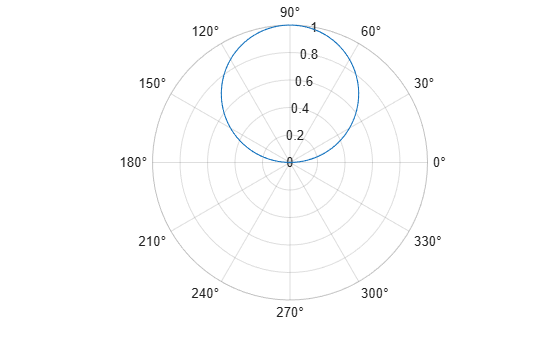Change the limits of the r-axis so it ranges from -1 to 1.

`rlim([-1 1])`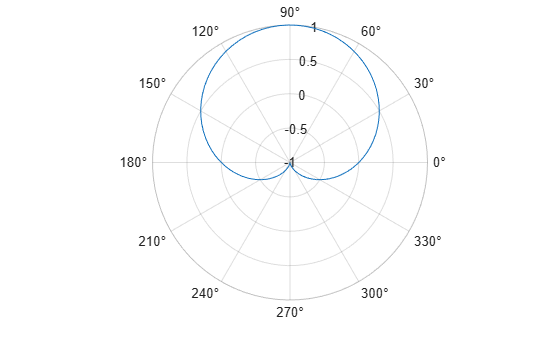Create a polar plot using a red line with circle markers.

```theta = linspace(0,2*pi,25); rho = 2*theta; polarplot(theta,rho,'r-o')```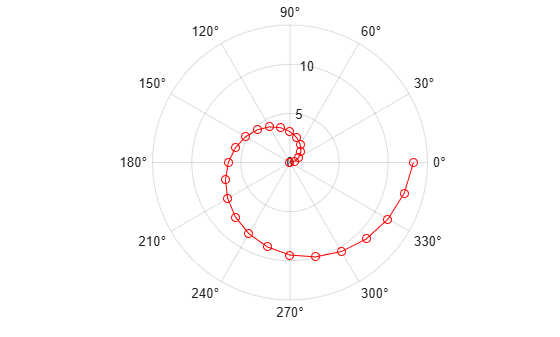Create a polar plot and return the chart line object.

```theta = linspace(0,2*pi,25); rho = 2*theta; p = polarplot(theta,rho);```Change the line color and width and add markers.

```p.Color = 'magenta'; p.Marker = 'square'; p.MarkerSize = 8;```Plot complex values in polar coordinates. Display markers at each point without a line connecting them.

```Z = [2+3i 2 -1+4i 3-4i 5+2i -4-2i -2+3i -2 -3i 3i-2i]; polarplot(Z,'*')```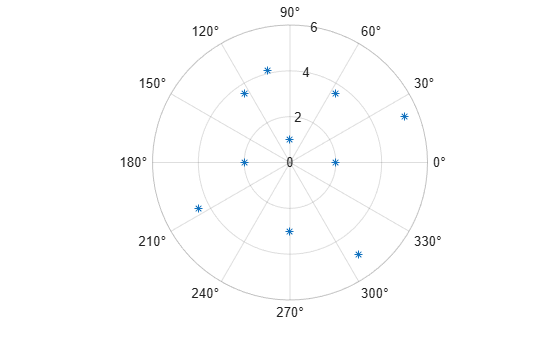## Input Arguments

collapse all

Angle values, specified as a vector or matrix. Specify the values in radians. To convert data from degrees to radians, use `deg2rad`.

To change the limits of the theta-axis, use `thetalim`.

Example: `[0 pi/2 pi 3*pi/2 2*pi]`

Radius values, specified as a vector or matrix. By default, negative values are reflected through 0. A point is reflected by taking the absolute value of its radius, and adding 180 degrees to its angle.

To change the limits of the r-axis, use `rlim`.

Example: `[1 2 3 4 5]`

Complex values, specified as a vector or matrix where each element is of the form `rho*`ei*theta, or `x+iy`, where:

• `rho = sqrt(x^2+y^2)`

• `theta = atan(y/x)`

Example: `[1+2i 3+4i 3i]`

Line specification, specified as a character vector or string containing a line style, marker, and color specifier. The elements can appear in any order, and you can omit one or more options. If you omit the line style and specify the marker, then the plot shows only markers with no line connecting them.

Example: `'--or'` is a red dashed line with circle markers

SpecifierLine Style
`-`Solid line (default)
`--`Dashed line
`:`Dotted line
`-.`Dash-dot line
SpecifierMarker
`o`Circle
`+`Plus sign
`*`Asterisk
`.`Point
`x`Cross
`s`Square
`d`Diamond
`^`Upward-pointing triangle
`v`Downward-pointing triangle
`>`Right-pointing triangle
`<`Left-pointing triangle
`p`Pentagram
`h`Hexagram
SpecifierColor

`y`

yellow

`m`

magenta

`c`

cyan

`r`

red

`g`

green

`b`

blue

`w`

white

`k`

black

`PolarAxes` object. You can modify the appearance and behavior of a `PolarAxes` object by setting its properties. For a list of properties, see PolarAxes Properties.

### Name-Value Pair Arguments

Specify optional comma-separated pairs of `Name,Value` arguments. `Name` is the argument name and `Value` is the corresponding value. `Name` must appear inside quotes. You can specify several name and value pair arguments in any order as `Name1,Value1,...,NameN,ValueN`.

Example: `'LineWidth',3`

`Name,Value` pair settings apply to all the lines plotted. You cannot specify different `Name,Value` pairs for each line using this syntax. Instead, return the chart line objects and use dot notation to set the properties for each line.

The properties listed here are only a subset. For a full list, see Line Properties.

Line color, specified as an RGB triplet, a hexadecimal color code, a color name, or a short name.

For a custom color, specify an RGB triplet or a hexadecimal color code.

• An RGB triplet is a three-element row vector whose elements specify the intensities of the red, green, and blue components of the color. The intensities must be in the range `[0,1]`; for example, ```[0.4 0.6 0.7]```.

• A hexadecimal color code is a character vector or a string scalar that starts with a hash symbol (`#`) followed by three or six hexadecimal digits, which can range from `0` to `F`. The values are not case sensitive. Thus, the color codes `'#FF8800'`, `'#ff8800'`, `'#F80'`, and `'#f80'` are equivalent.

Alternatively, you can specify some common colors by name. This table lists the named color options, the equivalent RGB triplets, and hexadecimal color codes.

Color NameShort NameRGB TripletHexadecimal Color CodeAppearance
`'red'``'r'``[1 0 0]``'#FF0000'``'green'``'g'``[0 1 0]``'#00FF00'``'blue'``'b'``[0 0 1]``'#0000FF'``'cyan'` `'c'``[0 1 1]``'#00FFFF'``'magenta'``'m'``[1 0 1]``'#FF00FF'``'yellow'``'y'``[1 1 0]``'#FFFF00'``'black'``'k'``[0 0 0]``'#000000'``'white'``'w'``[1 1 1]``'#FFFFFF'``'none'`Not applicableNot applicableNot applicableNo color

Here are the RGB triplets and hexadecimal color codes for the default colors MATLAB® uses in many types of plots.

RGB TripletHexadecimal Color CodeAppearance
`[0 0.4470 0.7410]``'#0072BD'``[0.8500 0.3250 0.0980]``'#D95319'``[0.9290 0.6940 0.1250]``'#EDB120'``[0.4940 0.1840 0.5560]``'#7E2F8E'``[0.4660 0.6740 0.1880]``'#77AC30'``[0.3010 0.7450 0.9330]``'#4DBEEE'``[0.6350 0.0780 0.1840]``'#A2142F'`Line style, specified as one of the options listed in this table.

Line StyleDescriptionResulting Line
`'-'`Solid line`'--'`Dashed line`':'`Dotted line`'-.'`Dash-dotted line`'none'`No lineNo line

Line width, specified as a positive value in points, where 1 point = 1/72 of an inch. If the line has markers, then the line width also affects the marker edges.

Marker symbol, specified as one of the markers in this table. By default, a chart line does not have markers. Add markers at each data point along the line by specifying a marker symbol.

ValueDescription
`'o'`Circle
`'+'`Plus sign
`'*'`Asterisk
`'.'`Point
`'x'`Cross
`'square'` or `'s'`Square
`'diamond'` or `'d'`Diamond
`'^'`Upward-pointing triangle
`'v'`Downward-pointing triangle
`'>'`Right-pointing triangle
`'<'`Left-pointing triangle
`'pentagram'` or `'p'`Five-pointed star (pentagram)
`'hexagram'` or `'h'`Six-pointed star (hexagram)
`'none'`No markers

Marker size, specified as a positive value in points, where 1 point = 1/72 of an inch.

Marker fill color, specified as `'auto'`, an RGB triplet, a hexadecimal color code, a color name, or a short name. The `'auto'` option uses the same color as the `Color` property of the parent axes. If you specify `'auto'` and the axes plot box is invisible, the marker fill color is the color of the figure.

For a custom color, specify an RGB triplet or a hexadecimal color code.

• An RGB triplet is a three-element row vector whose elements specify the intensities of the red, green, and blue components of the color. The intensities must be in the range `[0,1]`; for example, ```[0.4 0.6 0.7]```.

• A hexadecimal color code is a character vector or a string scalar that starts with a hash symbol (`#`) followed by three or six hexadecimal digits, which can range from `0` to `F`. The values are not case sensitive. Thus, the color codes `'#FF8800'`, `'#ff8800'`, `'#F80'`, and `'#f80'` are equivalent.

Alternatively, you can specify some common colors by name. This table lists the named color options, the equivalent RGB triplets, and hexadecimal color codes.

Color NameShort NameRGB TripletHexadecimal Color CodeAppearance
`'red'``'r'``[1 0 0]``'#FF0000'``'green'``'g'``[0 1 0]``'#00FF00'``'blue'``'b'``[0 0 1]``'#0000FF'``'cyan'` `'c'``[0 1 1]``'#00FFFF'``'magenta'``'m'``[1 0 1]``'#FF00FF'``'yellow'``'y'``[1 1 0]``'#FFFF00'``'black'``'k'``[0 0 0]``'#000000'``'white'``'w'``[1 1 1]``'#FFFFFF'``'none'`Not applicableNot applicableNot applicableNo color

Here are the RGB triplets and hexadecimal color codes for the default colors MATLAB uses in many types of plots.

RGB TripletHexadecimal Color CodeAppearance
`[0 0.4470 0.7410]``'#0072BD'``[0.8500 0.3250 0.0980]``'#D95319'``[0.9290 0.6940 0.1250]``'#EDB120'``[0.4940 0.1840 0.5560]``'#7E2F8E'``[0.4660 0.6740 0.1880]``'#77AC30'``[0.3010 0.7450 0.9330]``'#4DBEEE'``[0.6350 0.0780 0.1840]``'#A2142F'`## Tips

• To convert data from degrees to radians, use `deg2rad`. To convert data from radians to degrees, use `rad2deg`.

• You can modify polar axes properties to customize the chart. For a list of properties, see PolarAxes Properties.

• To plot additional data in the polar axes, use the ```hold on``` command. However, you cannot plot data that requires Cartesian axes in a polar chart.

Download ebook